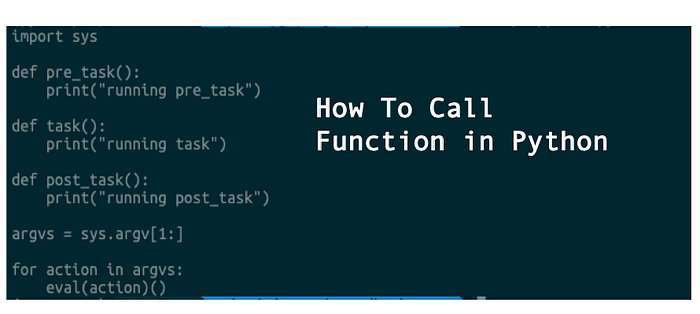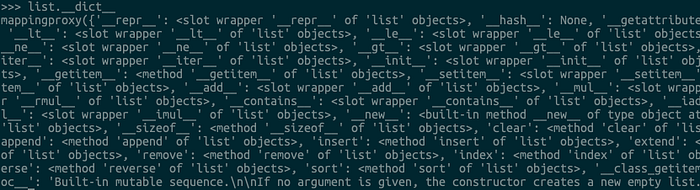# 9 Ways to Call a Function in Python

## How to call a function in Python?### 1. Directly Function Call

This is the simplest and most intuitive way:

``````def test():
print("This is a test")
test()
``````

### 2. Use partial() Function

In the built-in library of `functools`, there is a partial method dedicated to generating partial functions.

``````def power(x, n):
s = 1
while n > 0:
n = n - 1
s = s * x
return s

from functools import partial

power_2 = partial(power, n=2)
power_2(3)  # output: 9
power_2(4)  # output: 16
``````

### 3. Use eval()

If you need to execute the function dynamically, you can use eval + string to execute the function.

``````# demo.pyimport sys

argvs = sys.argv[1:]

for action in argvs:
eval(action)()
``````

To execute:

``````\$ python demo.py pre_task task post_task
``````

### 4. Use getattr()

If you put all the functions in the class and define them as static methods, you can use `getattr()` to get and call them.

``````import sys

@staticmethod

@staticmethod

@staticmethod

argvs = sys.argv[1:]

for action in argvs:
func()
``````

### 5. Use dict()

We all know that the object has a `__dict__()`magic method, it stores the properties and methods of any object. For example:You can call class method use `__dict__.get` :

``````import sys

@staticmethod

func.__func__()

# Output
\$ python /tmp/demo.py
``````

### 6. Use global()

``````import sys

argvs = sys.argv[1:]

for action in argvs:
globals().get(action)()

# Output
``````

### 7. Compile and Run From Text

You can define your function in a string, and use the `compile` function to compile it into byte code, and then used `exec` to execute it.

``````pre_task = """
"""
exec(compile(pre_task, '', 'exec'))# Or from a text file
with open('source.txt') as f:
exec(compile(source, 'source.txt', 'exec'))
``````

### 8. Use attrgetter()

In the built-in library of `operator`, there is a method for obtaining attributes, called `attrgetter`, execute after obtaining the function.

``````from operator import attrgetter

class People:
def speak(self, dest):
print("Hello, %s" %dest)

p = People()
caller = attrgetter("speak")
caller(p)("Tony")

# Output
\$ python /tmp/demo.py
Hello, Tony
``````

### 9. Use methodcaller()

There is also a `methodcaller` method in `operator` :

``````from operator import methodcaller

class People:
def speak(self, dest):
print("Hello, %s" %dest)

caller = methodcaller("speak", "Tony")
p = People()
caller(p)

# Output
\$ python /tmp/demo.py
Hello, Tony
``````

And these are the nine ways in which a function can be called in Python.